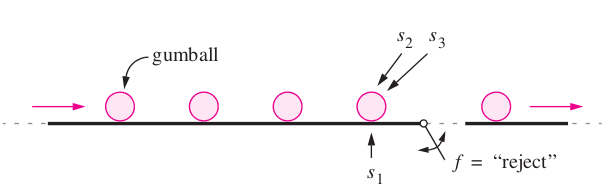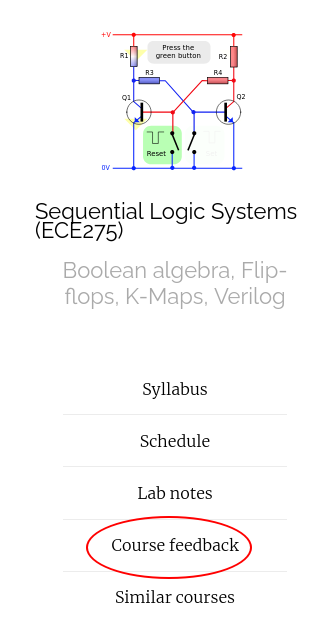## AnnouncmentsThe figure depicts a part of a factory that makes bubble gumballs. The gumballs travel on a conveyor that has three associated sensors $$s_1$$ , $$s_2$$ , and $$s_3$$. The sensor $$s_1$$ is connected to a scale that weighs each gumball, and if a gumball is not heavy enough to be acceptable then the sensor sets $$s_1 = 1$$. Sensors $$s_2$$ and $$s_3$$ examine the diameter of each gumball. If a gumball is too small to be acceptable, then $$s_2 = 1$$, and if it is too large, then $$s_3 = 1$$. If a gumball is of an acceptable weight and size, then the sensors give $$s_1 = s_2 = s_3 = 0$$. The conveyor pushes the gumballs over a “trap door” that it used to reject the ones that are not properly formed. A gumball should be rejected if it is too large, or both too small and too light. The trap door is opened by setting the logic function $$f$$ to the value 1.
Brown and Vranesic 2013, Example 2.7
• Find the minimial cost two-level circuit using K-maps (try both SOP/POS forms).
• Verify your answer using Quine McCluskey method (try only one of the forms).
• Try finding a lower cost implementation using functional decomposition with maximum fan-in of 2. Does functional decomposition lower the cost?
• Is the circuit hazard free? If not, design a Hazard free circuit.

## Practice problem

$f(x_1, \dots, x_4) = \sum m(0, 2, 4, 5, 7, 8, 9, 15)$
Brown and Vranesic 2013, Problem 8.18
• Find the minimial cost two-level circuit using K-maps.
• Try finding a lower cost implementation using functional decomposition with maximum fan-in of 2. Does functional decomposition lower the cost?
• Is the circuit hazard free? If not, design a Hazard free circuit.

## Design a 3-bit 1's complement circuit

that complements a 3-bit signed magnitude representation $$x_2 x_1 x_0$$

Design a Multi-output circuit that adds two 1-bit binary numbers $$x_0$$ and $$y_0$$ and outputs a sum and carry bit. $s_0 = (x_0 + y_0) \% 2$ $c_0 = ( x_0 + y_0 ) // 2$ where $$//$$ stands for Quotient and $$\%$$ for remainder.

Design a Multi-output circuit that adds three 1-bit binary numbers $$x_1$$, $$y_1$$ and $$c_0$$ outputs a sum and carry bit. $s_1 = ( x_1 \boxplus y_1 \boxplus c_0) \% 2$ $c_1 = ( x_1 \boxplus y_1 \boxplus c_0 ) // 2$ where $$//$$ stands for Quotient and $$\%$$ for remainder.

https://vikasdhiman.info/ECE275-Sequential-Logic/